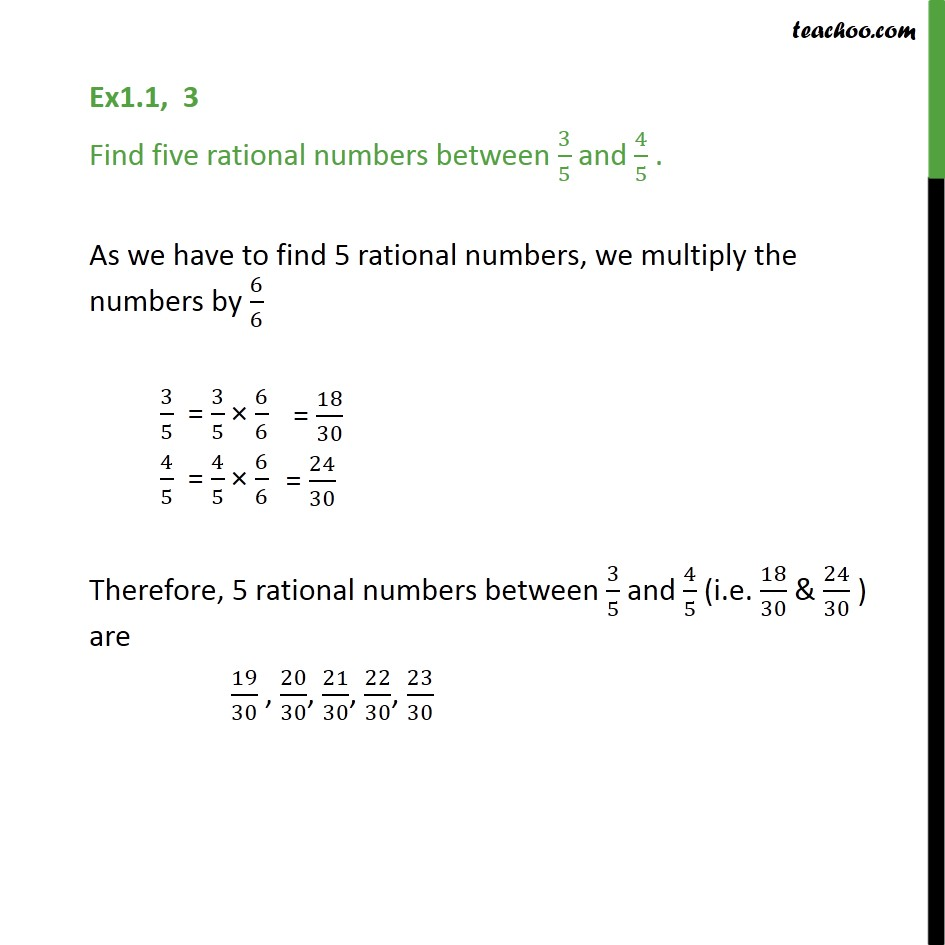Learn All Concepts of Chapter 1 Class 9 - FREE. Check - Chapter 1 Class 9 Maths1. Class 9
2. Important Questions for Exam - Class 9
3. Chapter 1 Class 9 Number Systems

Transcript

Ex1.1, 3 Find five rational numbers between 3/5 and 4/5 . As we have to find 5 rational numbers, we multiply the numbers by 6/6 3/5 = 3/5 × 6/6 4/5 = 4/5 × 6/6 Therefore, 5 rational numbers between 3/5 and 4/5 (i.e. 18/30 & 24/30 ) are 19/30 , 20/30, 21/30, 22/30, 23/30

Chapter 1 Class 9 Number Systems

Class 9
Important Questions for Exam - Class 9